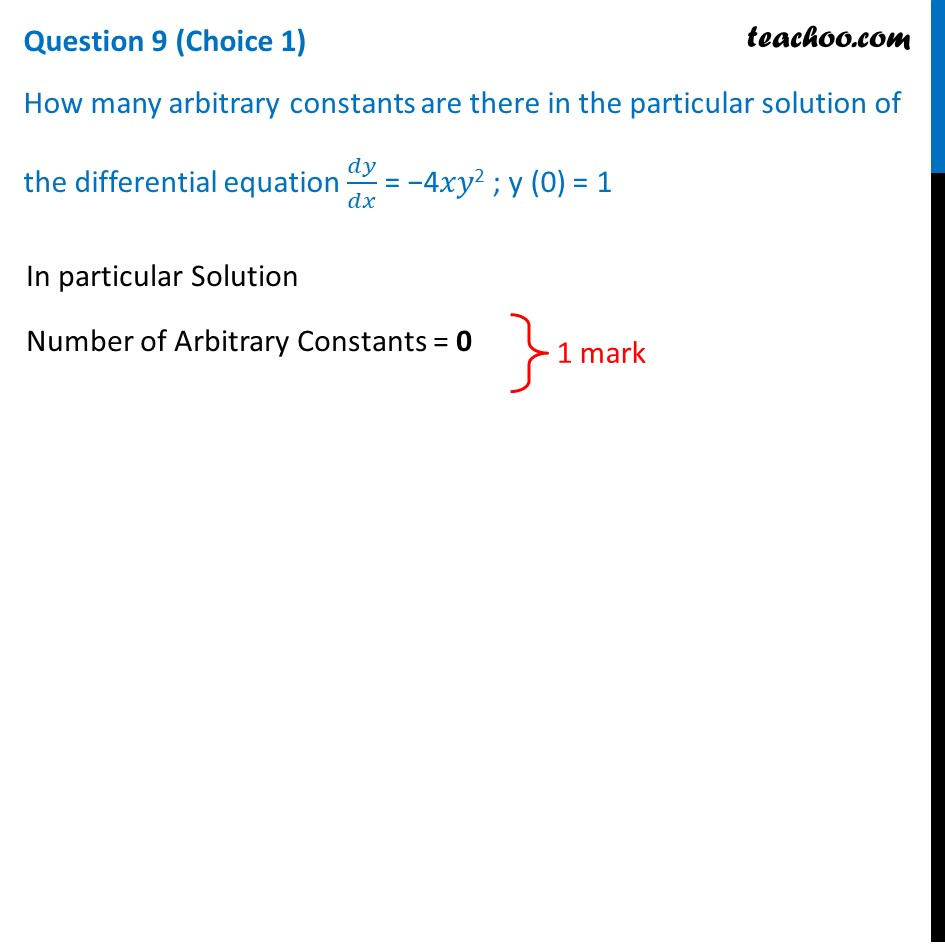## How many arbitrary constants are there in the particular solution of the differential equation dy/dx = −4𝑥𝑦 2 ; y (0) = 1Note : This is similar to Ex 9.2, 12 of NCERT – Chapter 9 Class 12 Differential Equations

1. Class 12
2. Solutions of Sample Papers and Past Year Papers - for Class 12 Boards
3. CBSE Class 12 Sample Paper for 2021 Boards

Transcript

Question 9 (Choice 1) How many arbitrary constants are there in the particular solution of the differential equation 𝑑𝑦/𝑑𝑥 = −4𝑥𝑦2 ; y (0) = 1 In particular Solution Number of Arbitrary Constants = 0

CBSE Class 12 Sample Paper for 2021 Boards

Class 12
Solutions of Sample Papers and Past Year Papers - for Class 12 Boards